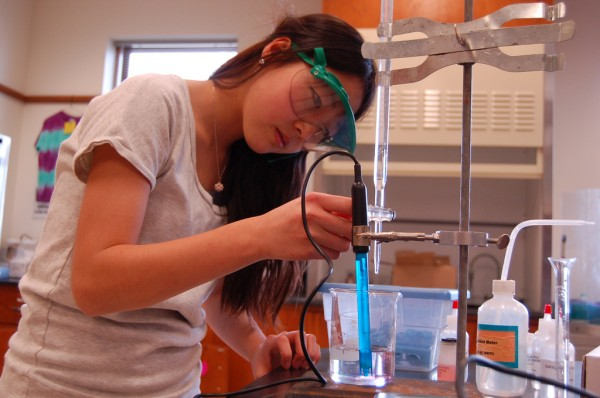## 9.3 Solution Stoichiometry

### Learning Objectives

By the end of this section, you will be able to:

• Perform stoichiometric calculations involving solution molarity

As we have seen in lab, many reactions such as single or double displacement reactions are carried out in aqueous medium (i.e. in water).  Because these reactions occur in aqueous solution, we can use the concept of molarity to directly calculate the number of moles of reactants or products that will be formed, and hence their amounts (i.e. volume of solutions or mass of precipitates).

More complex stoichiometry problems using balanced chemical reactions can also use concentrations as conversion factors (see example 3 below where the Ideal Gas Law is used in combination with solution stoichiometry). For example, suppose the following equation represents a chemical reaction:

$\large{\text{2 Ag}}{\text{NO}}_{3}\text{(}aq\text{)}{\text{ + CaCl}}_{2}\text{(}aq\text{)}\rightarrow {\text{2 AgCl}}\text{(}s\text{)}\text{ + Cu}{\text{(}{\text{NO}}_{3}\text{)}}_{2}\text{(}aq\text{)}$

If we wanted to know what volume of 0.555 M CaCl2 would react with 1.25 mol of AgNO3, we first use the balanced chemical equation to determine the number of moles of CaCl2 that would react and then use concentration to convert to liters of solution:

$\large1.25\cancel{\text{ mol Ag}{\text{NO}_{3}}}\times \frac{1\cancel{\text{ mol Ca}{\text{Cl}}_{2}}}{2\cancel{\text{ mol Ag}{\text{NO}_{3}}}}\times \frac{1\cancel{\text{ L solution}}}{0.555\cancel{\text{ mol Ca}{\text{Cl}_{2}}}}=\text{1.13}\text{ L Ca}{\text{Cl}_{2}}{\text{ solution}}$

This can be extended by starting with the mass (grams) of one reactant, instead of moles of a reactant.

### Example 1: Solution Stoichiometry–Mass to Volume Conversion

What volume of 0.0995 M Al(NO3)3 will react with 3.66 g of Ag according to the following chemical equation?

$\large{\text{3 Ag}}\text{(}s\text{)}\text{ + Al}{\text{(}{\text{NO}}_{3}\text{)}}_{3}\text{(}aq\text{)}\rightarrow {\text{3 Ag}}\text{NO}_{3}\text{(}aq\text{)}\text{ + Al}\text{(}s\text{)}$

What volume of 0.512 M NaOH will react with 17.9 g of H2C2O4(s) according to the following chemical equation?

$\large{\text{H}_{2}}{\text{C}}_{2}{\text{O}}_{4}\text{(}s\text{)}{\text{ + 2 NaOH}}\text{(}aq\text{)}\rightarrow {\text{Na}_{2}}{\text{C}}_{2}{\text{O}}_{4}\text{(}aq\text{)}\text{ + 2 H}_{2}\text{O}\text{(}l\text{)}$

We can extend our skills even further by recognizing that we can relate quantities of one solution to quantities of another solution. Knowing the volume and concentration of a solution containing one reactant, we can determine how much of another solution of another reactant will be needed using the balanced chemical equation.

### Example 2: Solution Stoichiometry–Volume to Volume Conversion

A student takes a precisely measured sample, called an aliquot, of 10.00 mL of a solution of FeCl3. The student carefully adds 0.1074 M Na2C2O4 until all the Fe3+(aq) has precipitated as Fe2(C2O4)3(s). Using a precisely measured tube called a burette, the student finds that 9.04 mL of the Na2C2O4 solution was added to completely precipitate the Fe3+(aq). What was the concentration of the FeCl3 in the original solution? (A precisely measured experiment like this, which is meant to determine the amount of a substance in a sample, is called a titration.) The balanced chemical equation is as follows:

$\large{\text{2 FeCl}_{3}}\text{(}aq\text{)}{\text{ + 3 Na}_{2}}\text{C}_{2}\text{O}_{4}\text{(}aq\text{)}\rightarrow {\text{ Fe}_{2}}{\text{(}{\text{C}}_{2}\text{O}_{4}\text{)}}_{3}\text{(}s\text{)}\text{ + 6 NaCl}\text{(}aq\text{)}$

A student titrates 25.00 mL of H3PO4 with 0.0987 M KOH. She uses 54.06 mL to complete the chemical reaction. What is the concentration of H3PO4?

$\large{\text{H}_{3}}{\text{PO}}_{4}\text{(}aq\text{)}{\text{ + 3 KOH}}\text{(}aq\text{)}\rightarrow {\text{K}_{3}}{\text{PO}}_{4}\text{(}aq\text{)}\text{ + 3 H}_{2}\text{O}\text{(}l\text{)}$### Example 3: Solution Stoichiometry & the IDeal GAs law

How many liters of carbon dioxide gas can form at STP when 125 mL of a 2.25 M HCl solution reacts with excess lithium carbonate?

$\large{\text{2 HCl}}\text{(}aq\text{)}{\text{ + Li}_{2}}\text{C}\text{O}_{3}\text{(}aq\text{)}\rightarrow {\text{C}}\text{O}_{2}\text{(}g\text{)}\text{ + 2 LiCl}\text{(}aq\text{)}{\text{ + H}_{2}}\text{O}\text{(}l\text{)}$

If 355 mL of hydrogen gas is collected at 25 oC at a total pressure of 740 mmHg from the reaction of excess zinc and a 3.0 M HCl solution, how many mL of the HCl solution was required?

$\large{\text{Zn}}\text{(}s\text{)}{\text{ + 2 HCl}}\text{(}aq\text{)}\rightarrow {\text{Zn}}{\text{Cl}}_{2}\text{(}aq\text{)}\text{ + H}_{2}\text{(}g\text{)}$

### Key Concepts and Summary

Molarity can be used as a conversion factor in combination with reaction stoichiometry.

### End of Module Problems

1.  Magnesium reacts with hydrochloric acid to produce hydrogen gas and magnesium chloride. How many liters of a 0.750 M HCl solution will react with 12.25 g of Mg?

$\large{\text{Mg}}\text{(}s\text{)}{\text{ + 2 HCl}}\text{(}aq\text{)}\rightarrow {\text{H}_{2}}\text{(}g\text{)}\text{ + Mg}{\text{Cl}}_{2}\text{(}aq\text{)}$

2.  Consider the following reaction:

$\large{\text{Pb}}\text{(}\text{N}\text{O}_{3}\text{)}_{2}\text{(}aq\text{)}{\text{ + 2 NaI}}\text{(}aq\text{)}\rightarrow {\text{PbI}_{2}}\text{(}s\text{)}\text{ + 2 NaN}{\text{O}}_{3}\text{(}aq\text{)}$

a.  How many grams of lead(II) iodide will be formed from 25.0 mL of a 2.00 M sodium iodide solution?

b.  How many milliliters of a 1.25 M lead(II) nitrate solution will react with 25.0 mL of a 1.50 M sodium iodide solution?

c.  What is the molarity of a 20.0 mL solution of sodium iodide that reacts completely with 60.0 mL of a 0.750 M lead(II) nitrate solution?

3.  Consider the following reaction:

$\large{\text{Al}_{2}}\text{(}\text{S}\text{O}_{4}\text{)}_{3}\text{(}aq\text{)}{\text{ + 6 KOH}}\text{(}aq\text{)}\rightarrow {\text{2 Al}}\text{(}\text{OH}\text{)}_{3}\text{(}s\text{)}\text{ + 3 K}_{2}{\text{SO}}_{3}\text{(}aq\text{)}$

a.  How many grams of aluminum hydroxide will be formed from 55.0 mL of a 1.50 M potassium hydroxide solution?

b.  How many milliliters of a 0.250 M aluminum sulfate solution will react with 10.0 mL of a 3.00 M potassium hydroxide solution?

c.  What is the molarity of a 40.0 mL solution of potassium hydroxide that reacts completely with 20.0 mL of a 0.500 M aluminum sulfate solution?

4. Copper(II) oxide reacts with hydrochloric acid to produce copper(II) chloride and water. How many liters of a 4.50 M HCl solution will react with 33.0 g of copper(II) oxide?

$\large{\text{CuO}}\text{(}s\text{)}{\text{ + 2 HCl}}\text{(}aq\text{)}\rightarrow {\text{CuCl}_{2}}\text{(}aq\text{)}\text{ + H}_{2}\text{O}\text{(}l\text{)}$